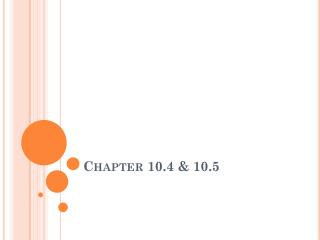DownloadDownload PresentationChapter 10.4 & 10.5

Chapter 10.4 & 10.5

Download PresentationChapter 10.4 & 10.5

- - - - - - - - - - - - - - - - - - - - - - - - - - - E N D - - - - - - - - - - - - - - - - - - - - - - - - - - -
Presentation Transcript

1. Chapter 10.4 & 10.5

2. 10.4 Using Inscribed Angles and Polygons • Inscribed angle- an angle whose vertex is on a circle and whose sides contain chords of the circle. • Intercepted arc- an arc that lies in the interior of an inscribed angle and has endpoints on the angle.

3. Measure of an inscribed angle theorem- The measure of an inscribed angle is one half the measure of its intercepted arc.

4. Example

5. Example

6. Theorem • If 2 inscribed angles of a circle intercept the same arc, then the angles are congruent.

7. Inscribed polygon- a polygon in which all the vertices lie on the circle • Circumscribed circle- the circle that contains the vertices of the polygon

8. Theorem • A quadrilateral can be inscribed in a circle if and only if its opposite angles are supplementary

9. Example • Find x and y.

10. Example • Find x and y.

11. Theorem • If a right triangle is inscribed in a circle, then the hypotenuse is a diameter of the circle. Conversely, if one side of an inscribed triangle is a diameter of the circle, then the triangle is a right triangle and the angle opposite the diameter is the right angle.

12. 10.5 Applying Other Angle Relationships in Circles • Theorem- • If a tangent and a chord intersect at a point on a circle, then the measure of each angle formed is one half the measure of its intercepted arc.

13. Intersecting Lines and Circles

14. Angles in the Circle Theorem • If the chords intersect inside a circle, then the measure of each angle is one half the sum of the measures of the arcs intercepted by the angle and its vertical angle

15. Angles Outside the Circle Theorem • If a tangent and a secant, 2 tangents, or 2 secants intersect outside a circle, then the measure of the angle formed is one half the difference of the measures of the intercepted arcs.